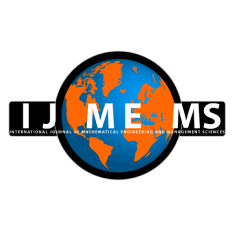### International Journal of Mathematical, Engineering and Management Sciences

#### ISSN: 2455-7749

Computationally Efficient Hybrid Method for the Numerical Solution of the 2D Time Fractional Advection-Diffusion Equation

#### Computationally Efficient Hybrid Method for the Numerical Solution of the 2D Time Fractional Advection-Diffusion Equation

School of Mathematical Sciences, Universiti Sains Malaysia, Penang, Malaysia.

Norhashidah Hj. Mohd Ali
School of Mathematical Sciences, Universiti Sains Malaysia, Penang, Malaysia.

;
Accepted on January 04, 2020

Abstract

In this paper, a hybrid method based on the Laplace transform and implicit finite difference scheme is applied to obtain the numerical solution of the two-dimensional time fractional advection-diffusion equation (2D-TFADE). Some of the major limitations in computing the numerical solution for fractional differential equations (FDEs) in multi-dimensional space are the huge computational cost and storage requirement, which are O(N^2) cost and O(MN) storage, N and M are the total number of time levels and space grid points, respectively. The proposed method reduced the computational complexity efficiently as it requires only O(N) computational cost and O(M) storage with reasonable accuracy when numerically solving the TFADE. The method’s stability and convergence are also investigated. The Results of numerical experiments of the proposed method are obtained and found to compare well with the results of existing standard finite difference scheme.

Keywords- Fractional advection-diffusion equation, Laplace transform, Finite difference scheme, Stability, Convergence.

Citation

Salama, F. M., & Ali, N. H. M. (2020). Computationally Efficient Hybrid Method for the Numerical Solution of the 2D Time Fractional Advection-Diffusion Equation. International Journal of Mathematical, Engineering and Management Sciences, 5(3), 432-446. https://doi.org/10.33889/IJMEMS.2020.5.3.036.

Conflict of Interest

The authors confirm that there is no conflict of interest to declare for this publication.

Acknowledgements

The authors extend their sincere appreciation to the editor and reviewers for their time and valuable suggestions. The authors also gratefully acknowledge the financial support from Universiti Sains Malaysia Research University Grants (1001/PMATHS/8011016).

References

Ali, U., Abdullah, F.A., & Mohyud-Din, S.T. (2017). Modified implicit fractional difference scheme for 2D modified anomalous fractional sub-diffusion equation. Advances in Difference Equations, 2017(1), 185. doi:10.1186/s13662-017-1192-4.

Balasim, A.T., & Ali, N.H.M. (2017). New group iterative schemes in the numerical solution of the two-dimensional time fractional advection-diffusion equation. Cogent Mathematics & Statistics, 4(1), 1412241.

Balasim, A.T. (2017). Fractional group iterative methods for two dimensional time-fractional differential equations. PhD Thesis. Universiti Sains Malaysia, Penang, Malaysia.

Bishehniasar, M., Salahshour, S., Ahmadian, A., Ismail, F., & Baleanu, D. (2017). An accurate approximate-analytical technique for solving time-fractional partial differential equations. Complexity, 2017, 1-12.

Chen, C.M., Liu, F., & Burrage, K. (2008). Finite difference methods and a Fourier analysis for the fractional reaction–subdiffusion equation. Applied Mathematics and Computation, 198(2), 754-769.

Gao, G.H., & Sun, H.W. (2015). Three-point combined compact difference schemes for time-fractional advection–diffusion equations with smooth solutions. Journal of Computational Physics, 298, 520-538.

Gong, C., Bao, W., Tang, G., Jiang, Y. & Liu, J. (2014). A parallel algorithm for the two-dimensional time fractional diffusion equation with implicit difference method. The Scientific World Journal, 2014, 1-8.

Gong, C., Bao, W., Tang, G., Jiang, Y., & Liu, J. (2015). Computational challenge of fractional differential equations and the potential solutions: a survey. Mathematical Problems in Engineering, 2015, 1-13.

Jiang, S., Zhang, J., Zhang, Q., & Zhang, Z. (2017). Fast evaluation of the Caputo fractional derivative and its applications to fractional diffusion equations. Communications in Computational Physics, 21(3), 650-678.

Lin, Y., & Xu, C. (2007). Finite difference/spectral approximations for the time-fractional diffusion equation. Journal of Computational Physics, 225(2), 1533-1552.

Mardani, A., Hooshmandasl, M.R., Heydari, M.H., & Cattani, C. (2018). A meshless method for solving the time fractional advection–diffusion equation with variable coefficients. Computers & Mathematics with Applications, 75(1), 122-133.

Miller, K.S., & Ross, B. (1993). An introduction to the fractional calculus and fractional differential equations. Wiley, New York.

Oldham, K.B., & Spanier, J. (1974). The Fractional Calculus. Academic Press, New York.

Podlubny, I. (1999). Fractional differential equations. Academic Press, New York.

Ren, J., Sun, Z.Z., & Dai, W. (2016). New approximations for solving the Caputo-type fractional partial differential equations. Applied Mathematical Modelling, 40(4), 2625-2636.

Samko, S.G., Kilbas, A.A., & Marichey, O.I. (1993). Fractional integrals and derivatives: theory and applications. Gordon & Breach, Yverdon.

Shen, S., Liu, F., & Anh, V. (2011). Numerical approximations and solution techniques for the space-time Riesz–Caputo fractional advection-diffusion equation. Numerical Algorithms, 56(3), 383-403.

Vong, S., Shi, C., & Lyu, P. (2018). A study on a second order finite difference scheme for fractional advection–diffusion equations. Numerical Methods for Partial Differential Equations, 35(2), 493-508.

Zhang, F., Gao, X., & Xie, Z. (2019). Difference numerical solutions for time-space fractional advection diffusion equation. Boundary Value Problems, 2019(1), 14.

Zhuang, P., Gu, Y., Liu, F., Turner, I., & Yarlagadda, P.K.D.V. (2011). Time‐dependent fractional advection–diffusion equations by an implicit MLS meshless method. International Journal for Numerical Methods in Engineering, 88(13), 1346-1362.

Zill, D.G. (2012). A first course in differential equations with modeling applications. Brooks Cole, Boston.